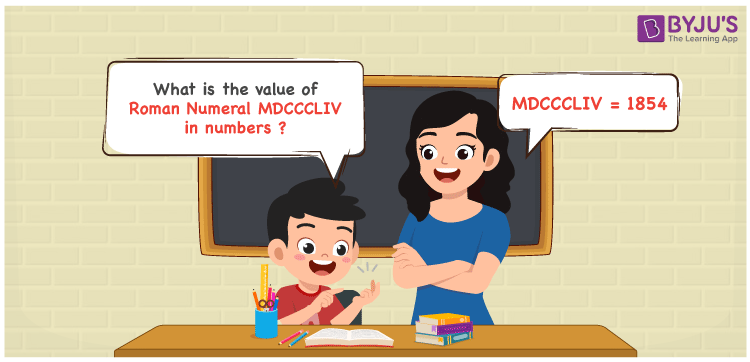Checkout JEE MAINS 2022 Question Paper Analysis : Checkout JEE MAINS 2022 Question Paper Analysis :

# MDCCCLIV Roman Numerals

MDCCCLIV Roman Numerals is 1854. This article shows the transformation of MDCCCLIV to numbers designed with care to assist students. The roman numerals conversion articles can be used as a reference material and learn the conversion accordingly. Therefore, the roman numerals MDCCCLIV is written as 1854 in numbers.

 Number Roman Numeral 1854 MDCCCLIV## How to Write MDCCCLIV Roman Numerals in Numbers?

This section guides students with a proper knowledge of the steps used to write MDCCCLIV in numbers.

MDCCCLIV = M + D + C + C + C + L + (V – I)

MDCCCLIV = 1000 + 500 + 100 + 100 + 100 + 50 + (5 – 1)

MDCCCLIV = 1854

## Video Lesson on Roman Numerals## Frequently Asked Questions on MDCCCLIV Roman Numerals

### Why MDCCCLIV is the roman numeral of the number 1854?

We know that

M is the roman numerals of 1000

DCCC is the roman numerals of 800

L is the roman numerals of 50

IV is the roman numerals of 4

So MDCCCLIV is the roman numeral of 1854.

### What is the value of 1900 – 46?

We know that

1900 – 46 = 1854

Hence, 1900 – 46 is 1854 which is written as MDCCCLIV.

### What is the remainder if MDCCCLIV is divided by XIX?

We know that

MDCCCLIV = 1854

XIX = 19

The remainder if MDCCCLIV is divided by XIX is XI.